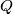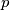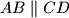### IMO Shortlist 1959 problem 6

Kvaliteta:
Avg: 0,0
Težina:
Avg: 0,0
Two planes,$P$ and$Q$, intersect along the line$p$. The point$A$ is given in the plane$P$, and the point$C$ in the plane$Q$; neither of these points lies on the straight line$p$. Construct an isosceles trapezoid$ABCD$ (with$AB \parallel CD$) in which a circle can be inscribed, and with vertices$B$ and$D$ lying in planes$P$ and$Q$ respectively.
Izvor: Međunarodna matematička olimpijada, shortlist 1959